Chapter 19, Problem 87GQ

Chapter
Section
Textbook Problem

Two Ag+(aq) | Ag(s) half-cells are constructed. The first has [Ag+] = 1.0 M, the second has [Ag+] = 1.0 × 10−5 M. When linked together with a salt bridge and external circuit, a cell potential is observed. (This kind of voltaic cell is referred to as a concentration cell.) (a) Draw a picture of this cell, labeling all components. Indicate the cathode and the anode, and indicate in which direction electrons flow in the external circuit. (b) Calculate the cell potential at 298 K.

(a)

Interpretation Introduction

Interpretation:

The picture of the cell, labeling all component has to be drawn and indicate cathode and the anode and indicate the direction in which electrons flow in the external unit.

Concept introduction:

Voltaic cell or Galvanic cell:

The device to produce electricity by using chemical reactions. In these divces are redox chemical reactions are occured.

A voltaic cell converts chemical energy into electrical energy.

It consists of two half cells. Each half cell consists of a metal and a solution of a salt of metal. Two half cells are connected by salt bridge.

The chemical reaction in the half cell is an oxidation reduction (redox)reactions.

For example:

Cell diagram of voltaic or galvanic cell is as follows.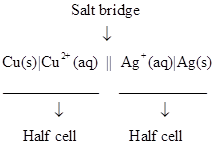According to the first law of thermodynamics, the change in internal energy of a system is equal ti the heat added to the sysytem minus the work done by the system.

The equation is as follows.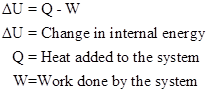In voltaic cell, the maximum cell potential is directly related to the free energy difference between the reactants and products in the cell.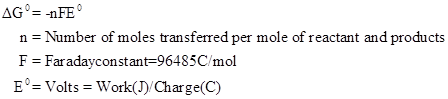From the relation, we can state that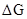value is negative. This means it is a spontaneous, the system spontaneously moves towards equilibrium.

The relation between standard cell potential and equilibrium constant is as follows.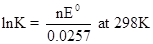The relation between solubility product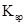and equilibrium constant is as follows.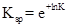Explanation

It consists of two half cells. Each half cell consists of a metal and a solution of a salt of metal. Two half cells are connected by salt bridge.

The chemical reaction in the half cell is an oxidation reduction (redox)reactions.

For the given information , Two half cells are constructed. The first has

(b)

Interpretation Introduction

Interpretation:

The cell potential athas to be calculated.

Concept introduction:

Voltaic cell or Galvanic cell:

The device to produce electricity by using chemical reactions. In these divces are redox chemical reactions are occured.

A voltaic cell converts chemical energy into electrical energy.

It consists of two half cells. Each half cell consists of a metal and a solution of a salt of metal. Two half cells are connected by salt bridge.

The chemical reaction in the half cell is an oxidation reduction (redox)reactions.

For example:

Cell diagram of voltaic or galvanic cell is as follows.According to the first law of thermodynamics, the change in internal energy of a system is equal ti the heat added to the sysytem minus the work done by the system.

The equation is as follows.In voltaic cell, the maximum cell potential is directly related to the free energy difference between the reactants and products in the cell.From the relation, we can state thatvalue is negative. This means it is a spontaneous, the system spontaneously moves towards equilibrium.

The relation between standard cell potential and equilibrium constant is as follows.The relation between solubility productand equilibrium constant is as follows.Still sussing out bartleby?

Check out a sample textbook solution.

See a sample solution

The Solution to Your Study Problems

Bartleby provides explanations to thousands of textbook problems written by our experts, many with advanced degrees!

Get Started

Find more solutions based on key concepts